Anzeige

# Lecture 1 steam turbines.ppt

25. Mar 2023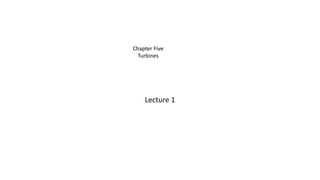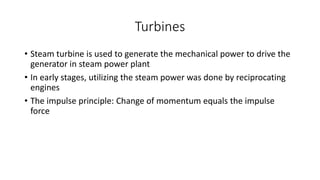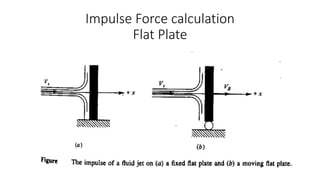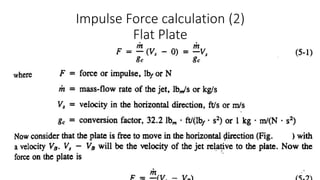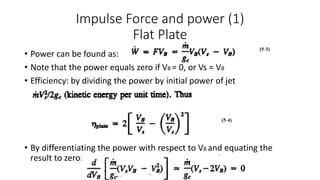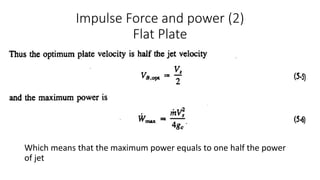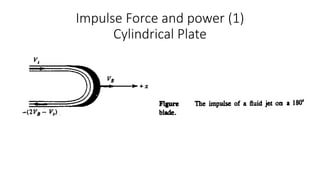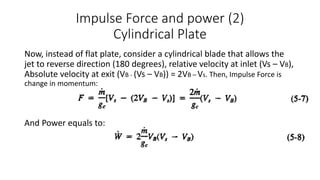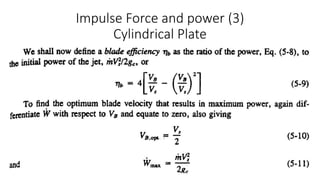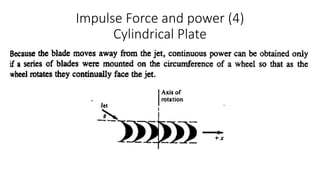1 von 10
Anzeige

### Lecture 1 steam turbines.ppt

1. Lecture 1 Chapter Five Turbines
2. Turbines • Steam turbine is used to generate the mechanical power to drive the generator in steam power plant • In early stages, utilizing the steam power was done by reciprocating engines • The impulse principle: Change of momentum equals the impulse force
3. Impulse Force calculation Flat Plate
4. Impulse Force calculation (2) Flat Plate
5. Impulse Force and power (1) Flat Plate • Power can be found as: • Note that the power equals zero if VB = 0, or Vs = VB • Efficiency: by dividing the power by initial power of jet • By differentiating the power with respect to VB and equating the result to zero:
6. Impulse Force and power (2) Flat Plate • W Which means that the maximum power equals to one half the power of jet
7. Impulse Force and power (1) Cylindrical Plate
8. Impulse Force and power (2) Cylindrical Plate Now, instead of flat plate, consider a cylindrical blade that allows the jet to reverse direction (180 degrees), relative velocity at inlet (Vs – VB), Absolute velocity at exit (VB - (Vs – VB)) = 2VB – Vs. Then, Impulse Force is change in momentum: And Power equals to:
9. Impulse Force and power (3) Cylindrical Plate
10. Impulse Force and power (4) Cylindrical Plate
Anzeige